# 1 Introduction to GenomicDistributions

If you have a set of genomic ranges, the GenomicDistributions R package can help you visualize properties of your region set. GenomicDistributions produces these nine types of plot:

• chromosome distribution plot - visualizes how your regions are distributed over chromosomes
• width distribution plot - visualizes the distribution of range widths
• feature distance distribution plot - visualizes how your regions are distributed in distance to the nearest feature of interest, like Transcription Start Sites (TSSs).
• partition distribution plot - visualizes how your regions are distributed across a genomic partitioning, such as frequency of overlapping a gene body, exon, promoter, intronic, or intergenic segment.
• signal in regions plot - visualizes signal of interest summary in a set of genomic ranges. In addition to your input genomic ranges, this plot type requires a data matrix with signal values for conditions of interest. As part of associated GenomicDistributionsData package, we have precompiled open chromatin signal matrices, which contain open chromatin signal values across genome from diverse set of cell types.
• neighboring regions distance - visualizes the distance between chromosomes neighboring regions.
• GC content plot - visualizes a probability density function of GC content percentage over the genomic ranges in the query.
• dinucleotide frequency - visualizes dinucleotide content within your regions.

GenomicDistributions can work with any reference genome, as long as you have some annotation data for it (like chromosome sizes and locations of genes). To make things easier for the common use cases, I’ve included in the package basic metadata for the most commonly used features from the reference genomes I use most (hg19, hg38, and mm10). If you need to produce similar plots with different features, partitions, or reference assemblies, that’s also possible, and not much more difficult; GenomicDistributions is very modular and will work with other bioconductor packages to process that data, but it requires one or two additional steps to curate your reference data (see the Custom reference and features section of this vignette).

In this vignette, we’ll go through examples of each of the plots using my common built-in features and partitions. If you want more control, there’s another advanced vignette that will introduce you how to define your own features, partitions, and chromosome sizes for custom analysis.

## 1.1 Philosophy of modular calc and plot functions

Before we start, I want to explain the design philosophy for functions in this package. Many R plotting packages combine calculations and plotting into one function. This may seem convenient, but if you want to plot the calculation results in a different way or combine them with something else, you can’t because you only have access to the final plot. GenomicDistributions divides these tasks so you can use the intermediate data to design your own custom plot, or use the calculated results directly for other analysis.

In GenomicDistributions, each plot type has two functions: a calculate function and a plot function. The calculate functions take your GRanges object and return a table of summary results. You can use these summary statistics how you like – aggregate them across multiple region sets, insert them into other plots you have, and so forth; or, you can simply plug that result directly into the corresponding plot function, which returns a ggplot2 object. Separating the calculation and plotting functions like this gives you more control over your results.

## 1.2 Installing GenomicDistributions

Install `GenomicDistributions` like this:

``````if (!requireNamespace("BiocManager", quietly = TRUE))
install.packages("BiocManager")
BiocManager::install("GenomicDistributions")``````

Or alternatively you can install from GitHub:

``devtools::install_github("databio/GenomicDistributions")``

Start by loading up the package and getting your query set of regions as a GenomicRanges object. I’ve included an example bed file to demonstrate how these plots look. You can load it up like this:

``````library("GenomicDistributions")
queryFile = system.file("extdata", "vistaEnhancers.bed.gz", package="GenomicDistributions")
query = rtracklayer::import(queryFile)``````

# 2 GenomicDistributions plot types

## 2.1 Chromosome distribution plots

Chromosome distribution plots help you visualize how your regions are distributed across chromosomes. To produce these, you’ll need to specify the chromosome lengths for your reference assembly. There are a few ways to do this.

For the common reference assemblies that I use (hg19, hg38, mm9, and mm10), I’ve included the metadata in the package. If you’re working with one of these genomes, making a plot of the distribution across chromosomes takes just a couple of lines of code:

``````# First, calculate the distribution:
x = calcChromBinsRef(query, "hg19")

# Then, plot the result:
plotChromBins(x)``````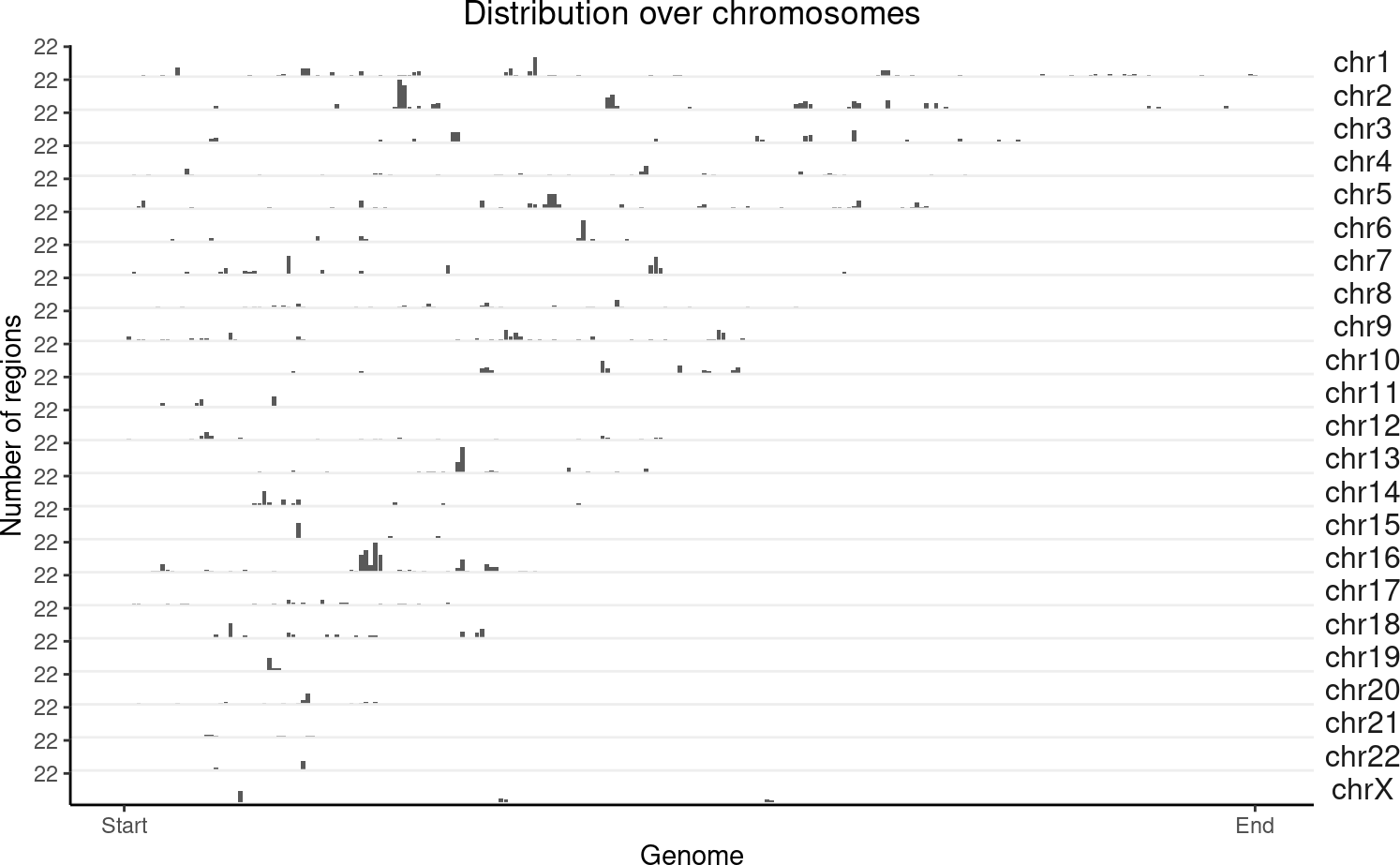What if we want to do the same thing but on 2 query sets at the same time? No problem:

``````# Let's fudge a second region set by shifting the first one over
query2 = GenomicRanges::shift(query, 1e6)
queryList = GRangesList(vistaEnhancers=query, shifted=query2)
x2 = calcChromBinsRef(queryList, "hg19")
plotChromBins(x2)``````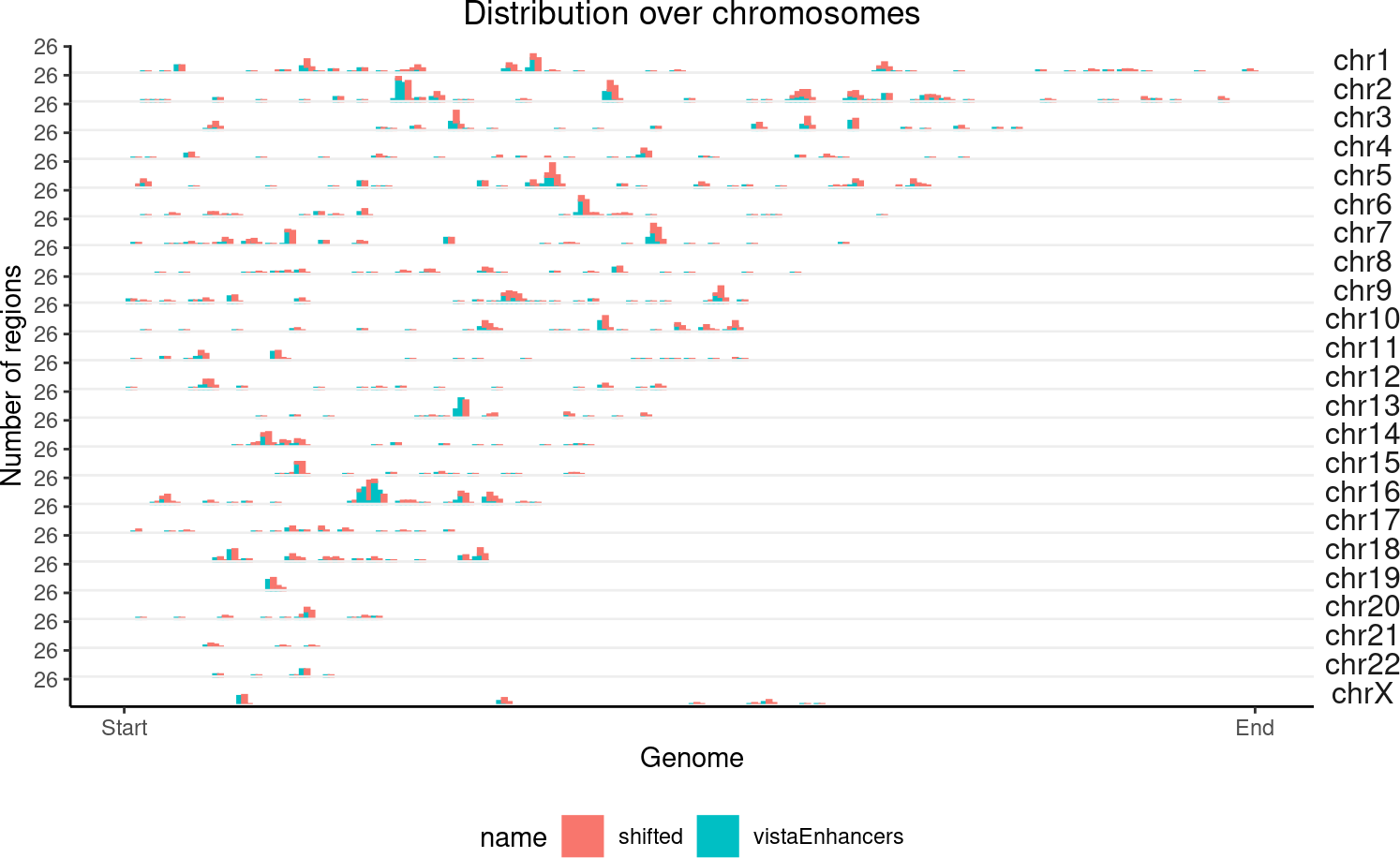These functions just do a naive binning across the genome. If you want to tweak the way the bins are handled, or use a different reference assembly, that’s also possible and is only slightly more complicated. There are other functions you can use for that, which are outlined in another vignette.

## 2.2 Feature distance distribution plots

Feature distance distribution plots will show you how your regions are distributed with respect to the nearest feature of interest. To illustrate, we’ll use Transcription Start Sites (TSS) as our example feature of interest (but really, you can use any region set).

For TSS plots, since this is such a common use case, we can use a handy built-in function that does everything for us. It’s just one line of code to check distances from query to your TSSs (for common genomes), and then a second line of code to plot those distances:

``````# Calculate the distances:
TSSdist = calcFeatureDistRefTSS(query, "hg19")

# Then plot the result:
plotFeatureDist(TSSdist, featureName="TSS")``````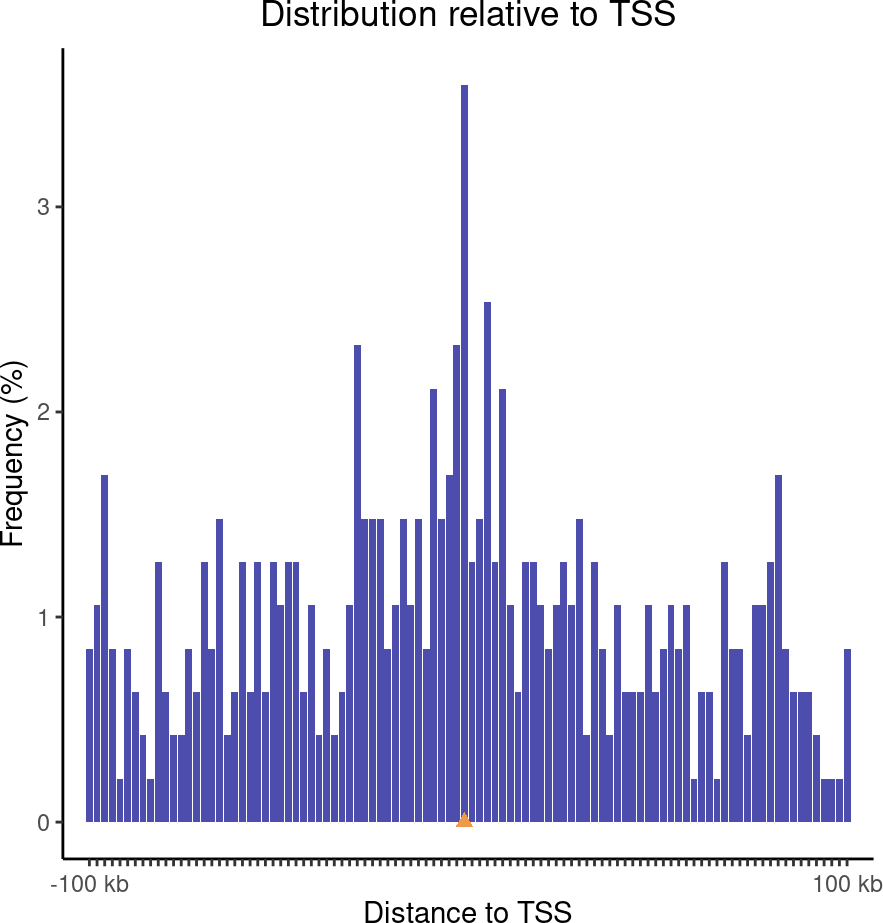Figure 1: TSS plot
Distribution of query regions relative to TSSs

This plot uses log-scale increasing bins to show how your regions are distributed. Now, let’s make a similar plot with multiple region sets input:

``````TSSdist2 = calcFeatureDistRefTSS(queryList, "hg19")
plotFeatureDist(TSSdist2, featureName="TSS")``````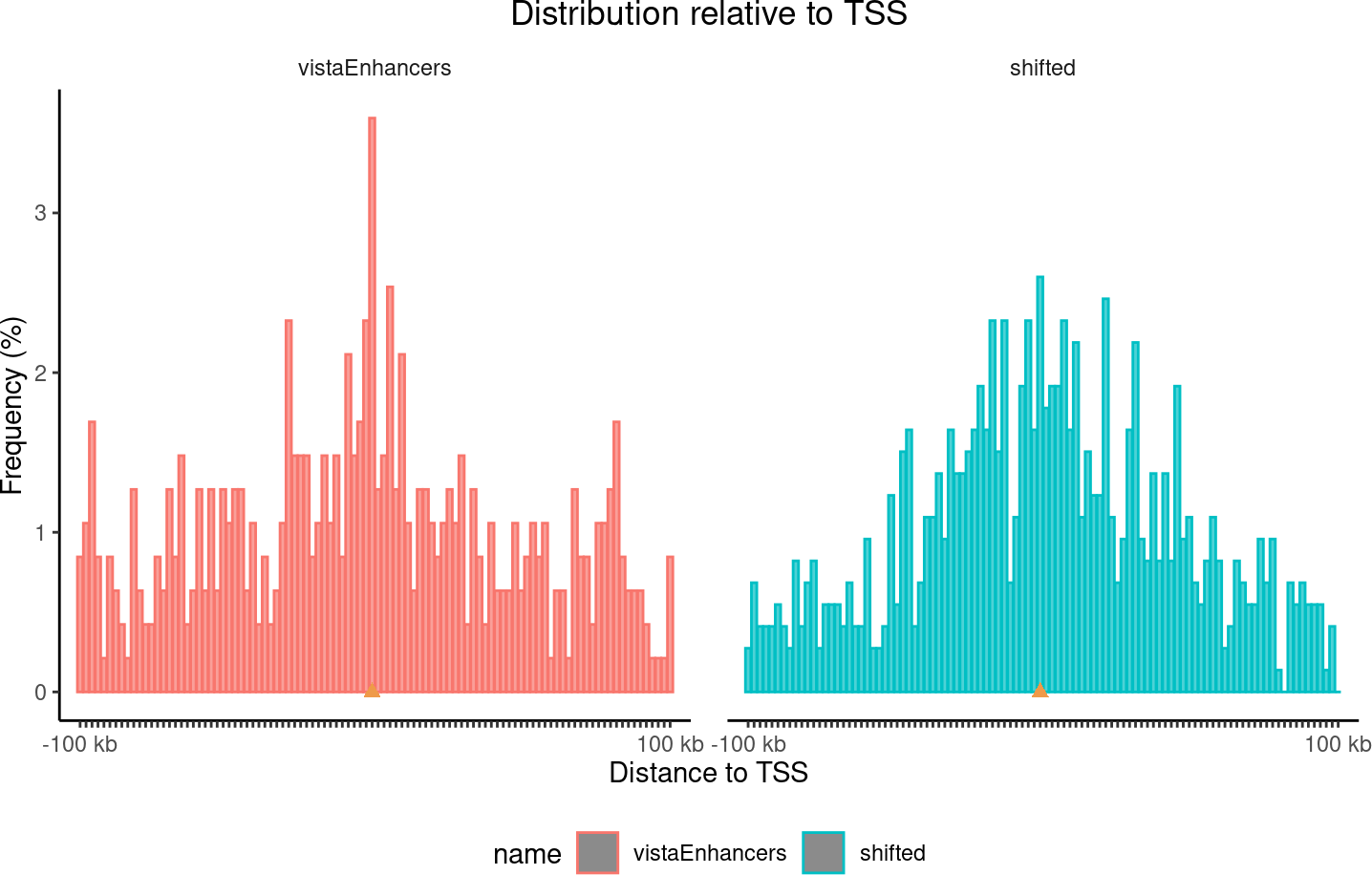Figure 2: TSS plots with multiple region sets

You can also plot a tiled version that aligns them all vertically, and you can sort the datasets based on the values in the center:

``plotFeatureDist(TSSdist2, featureName="TSS", tile=TRUE, labelOrder = "center")``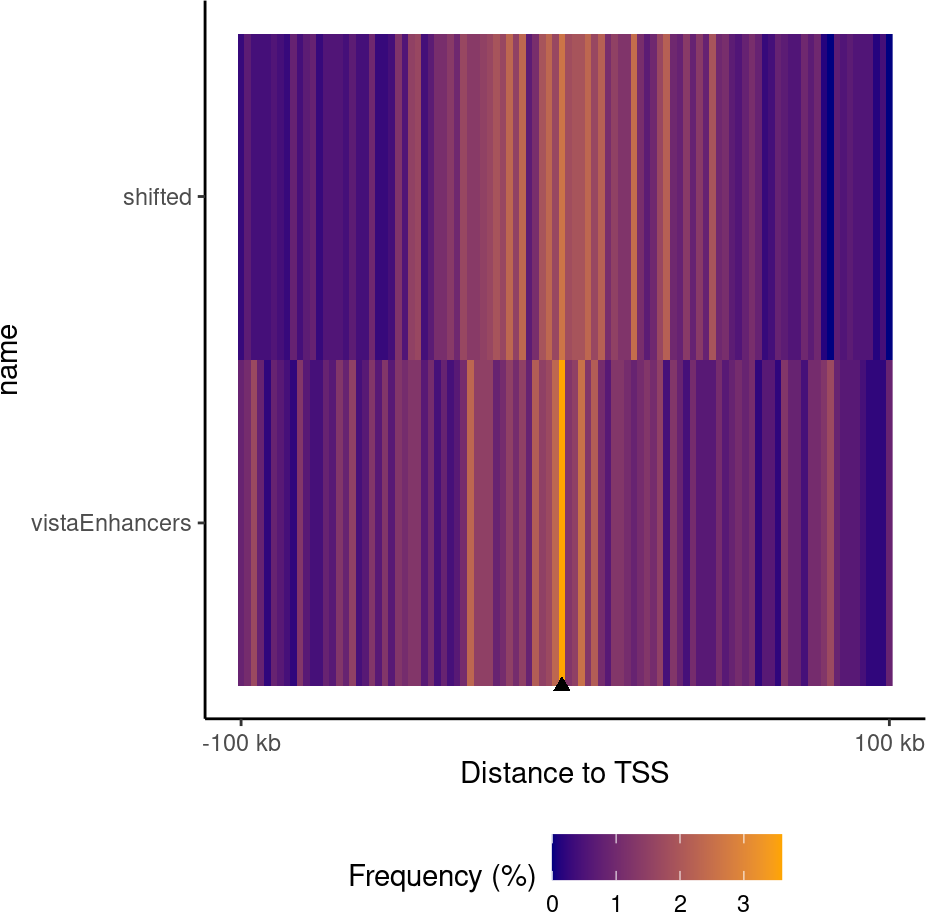Figure 3: Tiled feature distance plot
Distribution of query regions relative to arbitrary features

If you want to check distances to other features, that’s no problem; `calcFeatureDistRefTSS()` is really just a wrapper for the workhorse function, `calcFeatureDist()`. To show how this works, get some features you want to check the distance to. Here, let’s just shift our query set by a normally distributed random number:

``featureExample = GenomicRanges::shift(query, round(rnorm(length(query), 0,1000)))``

Now, with these features, we just use the `calcFeatureDist` function to calculate the distances. This function uses the fast rolling joins from `data.table` under the hood, so it completes very quickly. The result of this gets piped right into the plotting function as before:

``````fdd = calcFeatureDist(query, featureExample)
plotFeatureDist(fdd)``````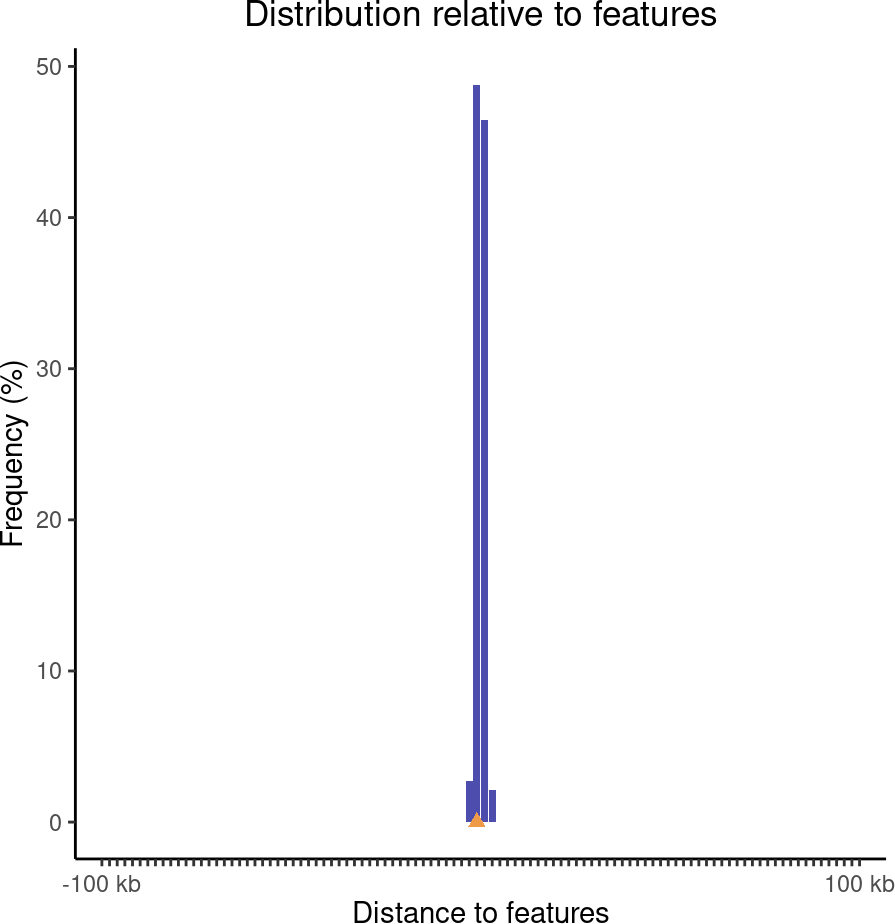Figure 4: Feature distance plot
Distribution of query regions relative to arbitrary features

## 2.3 Partition distribution plots

Genomic partition distribution plots show you how your regions are distributed across genome annotation classes. This is most commonly used to show the distribution over element types, such as promoters, exons, introns, or intergenic regions. GenomicDistributions provides 3 types of partition distribution plots: percentages, expected, and cumulative.

### 2.3.1 Percentage partition distribution plots

The most basic partition plot just provides a barplot of percentage region overlaps. You can produce one or two-set plots like so:

``````gp = calcPartitionsRef(query, "hg19")
plotPartitions(gp)``````